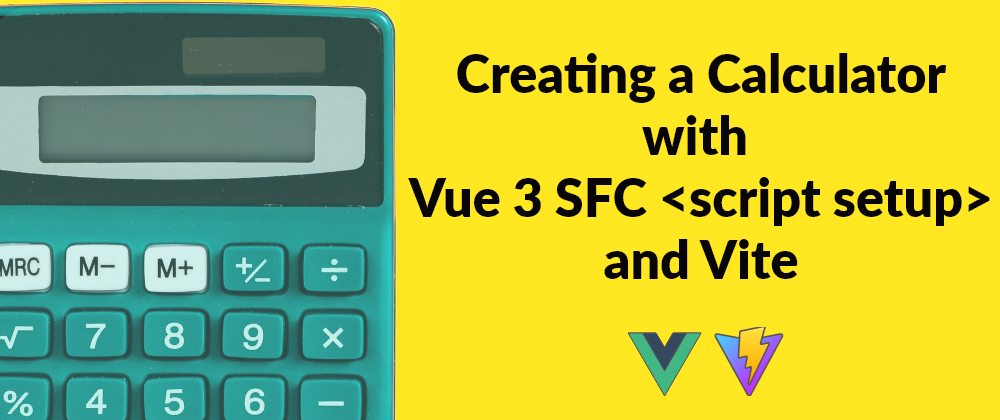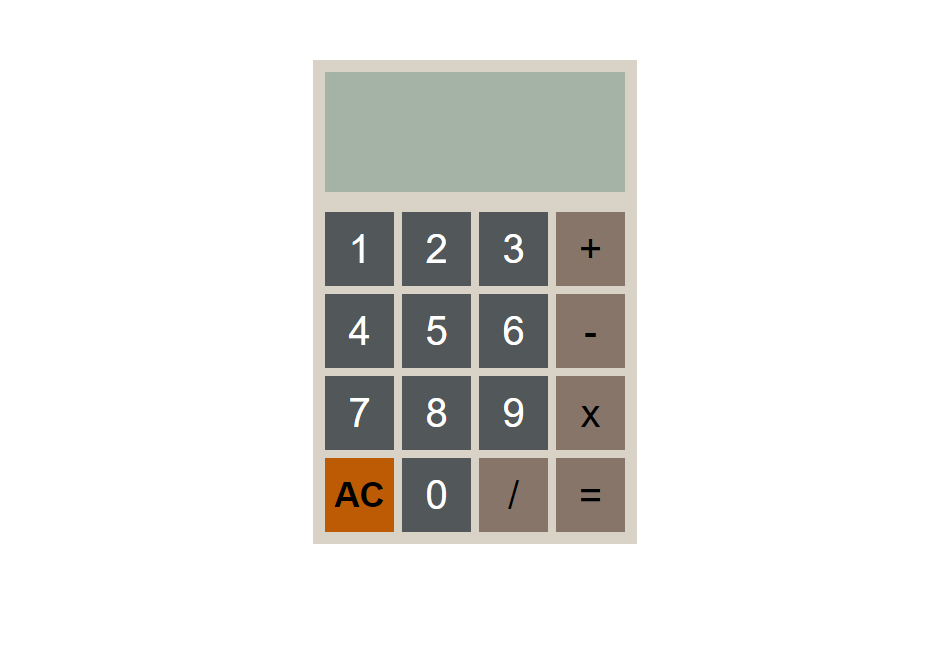# Creating a Calculator with Vue 3 SFC <script setup> and Vite

Oct 1, 2021Updated on Jan 7, 2022Vue's SFC `<script setup>` is a compile-time syntactic sugar for using Composition API inside Single File Vue Components (SFCs). This sugar comes with a number of advantages compared to the normal `<script>` syntax such as better runtime performance and enabling us to write concise code with less boilerplate. Here are the docs for more on this setup.

On this tutorial we are going to create a basic calculator with Vue's SFC `<script setup>` syntax to see it in action in a real world example. The source code for this calculator can also be found on this github repository.

The calculator we will be building will contain only four basic mathematical operations that are addition, subtraction, multiplication and division.

Let's get our hands dirty.

## Setting up the Project

In this project we are going to use Vite as our build tool leveraging it's fast and lean set up to ensure a smooth development experience. Start by creating a new Vite project using the Vue template. Do this by running the following script on your target directory.

``````npm init vite@latest vuelculator -- --template vue
``````

An important note while running this script at least in a windows machine is that, the path to the project's folder should not have a space in between otherwise you are going to experience an error. Here is one of the workarounds to fix this.

When the above script terminates, cd into the created project directory. The project's file setup will minimally be as follows:

``````.
├── src
|   └── components
|       └── HelloWorld.vue
|   └── App.vue
|   └── main.js
index.html
package.json
``````

Since we won't have any use for the `Helloworld.vue` component, delete it and remove its import from our root Vue component `App.vue`.

When you open the `App.vue` file you'll notice that the script tag contains the `setup` attribute, the `HelloWorld.vue` component was imported and made available to our template by just using an import statement. This is one of the advantages of the `script setup` sugar at work.

``````<script setup>
import Helloworld from './components/HelloWorld.vue'
</script>
``````

You do not need to add an imported child's instance to the parent component's `components` property to be able to use it in the parent's template since top-level bindings such as variables, imports and functions are exposed to the template. Just import the child component or add the function or variable and you can use it inside the template.

The code inside the `<script setup>` is handled just as the code inside the `setup()` function would be, but in addition to the later it is executed each time an instance of the component is created, contrast to the `setup()` function which executes once when the component is first imported.

For all the advantages this sugar carries over the normal `<script>` syntax the `<script setup>` is the recommended syntax when using Single File Components and the Composition API.

## The UI

First create a new component called `Calculator.vue` and place it in the `components` folder. Proceed to importing the component in the root `App.vue` file.

``````<!-- App.vue -->
<script setup>
import Calculator from './components/Calculator..vue'
</script>

<template>
<Calculator/>
</template>
``````

An important note when importing Vue SFCs within the Vue 3 + Vite setup is, DO NOT forget to include the `.vue` extension on the SFC file's name, otherwise you will get an import error.

Inside the `Calculator.vue` file, start with laying out the calculator's template. The two essential parts of the calculator are the display and keypad sections. We'll harness the power of CSS grid to have as little HTML boilerplate as possible while getting a presentable calculator nonetheless.

``````<template>
<h1>Vuelculator</h1>
<div class="calc">
<div class="display">
[[ equation ]]
</div>

<div class="key num">1</div>
<div class="key num">2</div>
<div class="key num">3</div>
<div class="key fn">+</div>

<div class="key num">4</div>
<div class="key num">5</div>
<div class="key num">6</div>
<div class="key fn">-</div>

<div class="key num">7</div>
<div class="key num">8</div>
<div class="key num">9</div>
<div class="key fn">x</div>

<div class="key special">AC</div>
<div class="key num">0</div>
<div class="key fn">/</div>
<div class="key fn">=</div>

</div>
</div>
</template>
``````

Then style that layout with some CSS.

``````...
.calc{
width: 320px;
height: 480px;
display: flex;
flex-direction: column;
margin-left: auto;
margin-right: auto;
background-color: #D9D3C7;
border: 2px solid #D9D3C7;
}

.display{
flex: 1;
background-color: #A5B3A6;
margin: 10px;
font-size: 40px;
text-align: right;
overflow-wrap: break-word;
}

height: 320px;
display: grid;
grid-template-columns: repeat(4, 1fr);
gap: 8px;
margin: 10px;
}

.key{
display: flex;
justify-content: center;
align-items: center;
font-size: 40px;
cursor: pointer;
}
.num{
background-color: #525759;
color: #ffffff;
}
.fn{
background-color: #877569;
color: #000000;
}
.special{
background-color: #BD5A04;
color: #000000;
font-size: 35px;
font-weight: bold;
}
::selection{
background: none;
}
...
``````

This will give us a calculator with the following look.## The Logic

Proceeding with the calculator, it is important to first prescribe what our calculator does. As we can see from the designed UI, this calculator has four basic mathematical operators (+, -, *, /), an All Clear button (AC), an equation processing "result" button (=) and the keys buttons, these will in total equate to roughly 7 functions.

Modify the UI by attaching the functions to their respective buttons.

``````...

<div class="key num" v-for="key in [1,2,3]"
@click="useNumber(key)">[[key]]</div>
<div class="key fn" @click="plus()">+</div>

<div class="key num" v-for="key in [4,5,6]"
@click="useNumber(key)">[[key]]</div>
<div class="key fn" @click="minus()">-</div>

<div class="key num" v-for="key in [7,8,9]"
@click="useNumber(key)">[[key]]</div>
<div class="key fn" @click="multiply()">x</div>

<div class="key special" @click="clear()">AC</div>
<div class="key num" @click="useNumber(0)">0</div>
<div class="key fn" @click="divide()">/</div>
<div class="key fn" @click="result()">=</div>

</div>
...
``````

Proceed with implementing the calculator's logic.

First, declare three reactive variables, equation which will be holding the equation String to be calculated and it's resulting answer to be shown on the display, lastResult which will be storing the result of the last calculation and resultCalled which will be storing the state of each result processing call.

``````  import {ref} from 'vue'

let equation = ref('0')
let resultCalled = ref(false);
let lastResult = ref(0);
``````

Place the equation variable on the display body so that we can see the formulated equation and resulting answers on our calculator.

``````...
<div class="display">
[[ equation ]]
</div>
...
``````

Next, declare the function that will be called when the number keys will be pressed. This function will be concatenating the number passed as its argument to the existing equation in real-time as we would have on a real calculator. It will also be checking the state of the equation and reacting accordingly. Name this function useNumber()

``````  const useNumber = (num) => {
equation.value = resultCalled.value ? num : equation.value.search(/^0/g) ? equation.value + num : (equation.value.search(/^[-]\$/g) !== -1 ? equation.value + num : num);
resultCalled.value = false;
};
``````

Afterwards, declare the functions called when the four different mathematical operator buttons are pressed.

``````  const plusOperator = ' + ';
const plus = () => {
equation.value = checkOperator(equation.value, plusOperator);
}
const minusOperator = ' - ';
const minus = () => {
equation.value = checkOperator(equation.value, minusOperator);
}
const multiplyOperator = ' x ';
const multiply = () => {
equation.value = checkOperator(equation.value, multiplyOperator);
}
const divideOperator = ' / ';
const divide = () => {
equation.value = checkOperator(equation.value, divideOperator);
}
``````

As seen from the code above these functions call a checkOperator() function that sanitizes the current equation before adding the operator to it. It checks whether the equation is at an initial state, another operator was added last or if a result was just processed and reacts accordingly.

``````  const checkOperator = (equation, requestedOperator) => {
if(equation.search(/^0\$/g) !== -1){
if(requestedOperator.search(/( [/x] )\$/g) !== -1) return '0';
else return requestedOperator.replace(/ /g, '')
}else{
if(resultCalled.value){
resultCalled.value = false;
return lastResult.value + requestedOperator;
}else{
return equation.replace(/( [+\-/x] )\$/g, '') + requestedOperator;
}
}
}
``````

Continue with adding the result calculating function - `result()` that takes the formulated equation, a `String`, and gives us a mathematical sound answer.

There are many ways to go about this, one of which is using the eval() JavaScript function, which if not for it's vulnerabilities would be a sound solution. But we'll use it's safe alternative shown below.

``````  const result = () => {
let finalEqn = equation.value.replace(/( [+\-/x] )\$/g, '')
resultCalled.value = finalEqn.search(/( [+\-/x] )/g) !== -1
let eqResult = Function('"use strict";return (' + finalEqn.replace(/( \x+ )/g, ' * ') + ')')();
equation.value = `\${eqResult.toLocaleString()}`;
lastResult.value = eqResult;
}
``````

Above, we update the state of the `resultCalled`, process the equation and assign the resulting answer into the `equation` variable so that it can be displayed on the calculator's display and finalize by storing the answer into `lastResult`.

Finish the logic part by adding the "All Clear" (AC) function which simply assigns the String `'0'` to the `equation`'s value.

``````  const clear = () => equation.value = '0'
``````

Bringing all the logic together we have the following script.

``````<script setup>
import { ref } from 'vue';

const equation = ref('0');
const useNumber = (num) => {
equation.value = resultCalled.value ? num : equation.value.search(/^0/g) ? equation.value + num : (equation.value.search(/^[-]\$/g) !== -1 ? equation.value + num : num);
resultCalled.value = false;
};
const plusOperator = ' + ';
const plus = () => {
equation.value = checkOperator(equation.value, plusOperator) + plusOperator;
}
const minusOperator = ' - ';
const minus = () => {
equation.value = checkOperator(equation.value, minusOperator) + minusOperator;
}
const multiplyOperator = ' x ';
const multiply = () => {
equation.value = checkOperator(equation.value, multiplyOperator) + multiplyOperator;
}
const divideOperator = ' / ';
const divide = () => {
equation.value = checkOperator(equation.value, divideOperator) + divideOperator;
}
const clear = () => equation.value = '0'
const checkOperator = (equation, requestedOperator) => {
if(equation.search(/^0\$/g) !== -1){
if(requestedOperator.search(/( [/x] )\$/g) !== -1) return '0';
else return requestedOperator.replace(/ /g, '')
}else{
if(resultCalled.value){
resultCalled.value = false;
return lastResult.value + requestedOperator;
}else{
return equation.replace(/( [+\-/x] )\$/g, '') + requestedOperator;
}
}
}
const result = () => {
let eqResult = Function('"use strict";return (' + equation.value.replace(/( \x+ )/g, ' * ') + ')')();
equation.value = eqResult;
}
</script>
``````

That's all for our basic calculator in Vue 3 script setup SFC + Vite. You can proceed to adding as many mathematical operations as possible into it by tweaking its UI and logic.

For a bit advanced version containing negative number operations to this calculator head to its github repo. I will be adding more mathematical operators to this calculator in time, feel free to fork and modify it to your liking.

Get creative and make outstanding calculators.